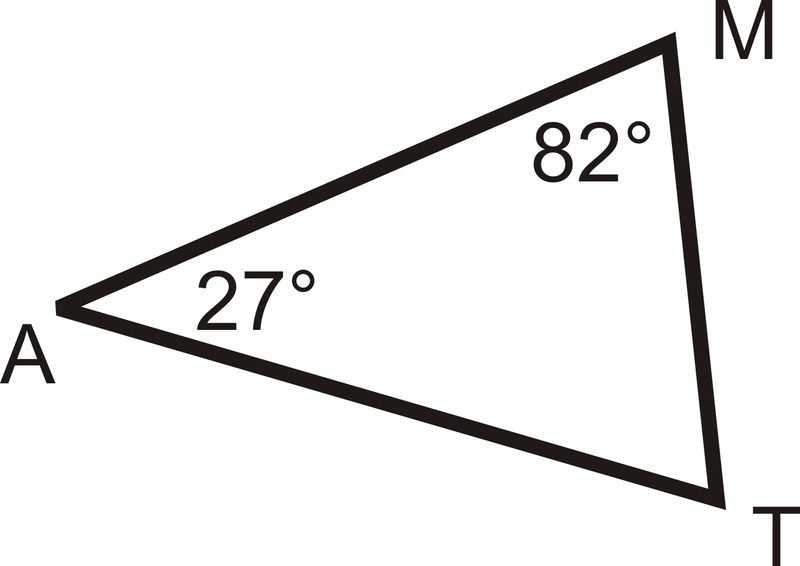Is It A Triangle?
Find a Third Side Range
Ordering Angles
Ordering Sides
100

Is it a triangle?

8, 18, 24

Is it a triangle?

Yes!

8+18>24

100

2 and 10

8 < x < 12

100

Order from least to greatestBC, AB, AC

100

Order from least to Greatest<P, <Q, <R

200

Is it a triangle?

28, 50, 90

Is it a triangle?

No!

28+50>90

200

3 and 5

2 < x < 8

200

Order from Greatest to leastJK, JL, LK

200

Order from Least to Greatest<R, <P, <Q

300

Is it a triangle?

41, 7, 55

Is it a triangle?

No!

7+41>55

300

7 and 7

0 < x < 14

300

Order Greatest to leastAC, BC, AB

300

Order from greatest to least<C, <B, <A

400

33°, 38°, 114°

No!

33+38+144 = 185

(It is not equal to 180)

400

If a triangle has lengths of 30 cm and 10 cm, what are all possible lengths for the third side? (not negatives, its to separate options)

- 10 cm

- 21 cm

- 30 cm

20 < x < 40

- 21 cm

- 30 cm

400

Order from least to greatestDE, EV, DV

400

Order least to greatest, the perimeter is 200<C, <B, <A

7x+10x+x=200

18x=200

x=11.11

500

34°, 34°, 112°

Yes!

34 + 34 + 112 = 180

(It is equal to 180)

500

If a triangle has the lengths of 16ft and 28 ft, what are all lengths of the possible side? (not negatives, its to separate options)

- 34 ft

- 12 ft

- 29 ft

- 44 ft

12 < x < 44

- 34 ft

- 29 ft

500

Order least to greatestMT, AM, AT

500

Order from greatest to least, the perimeter 200

AB                                                   C

<B, <A, <C

21x+15x+x= 200

37x=200

x= 5.4

Click to zoom